# AP化学的考题长什么样子？（II）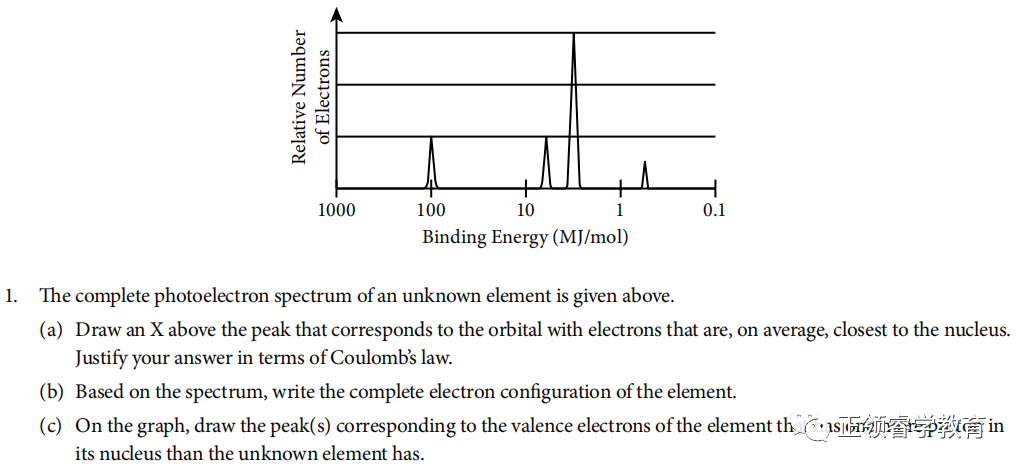(a)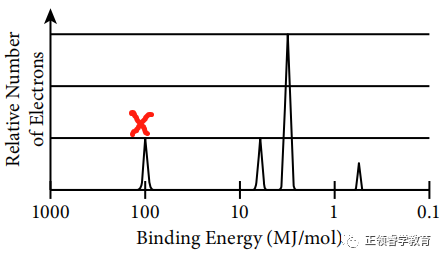Electrons closest to the nucleus have the greatest binding energy because according to Coulomb’s Law, the strength of attraction between the charges is greatest when the distance between them is the least.

(b) Based on spectrum, the electronic configuration is 1s22s22p63s1.

(c)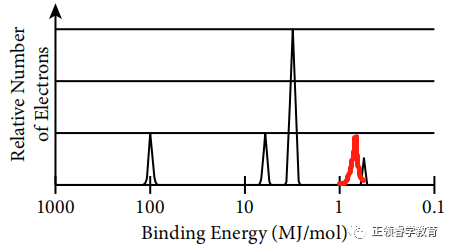One more proton in nucleus leads to stronger attraction to valence electrons so the binding energy is greater (to the left), and there are two valence electrons in this element; so the height of peak is also twice as that of the unknown element.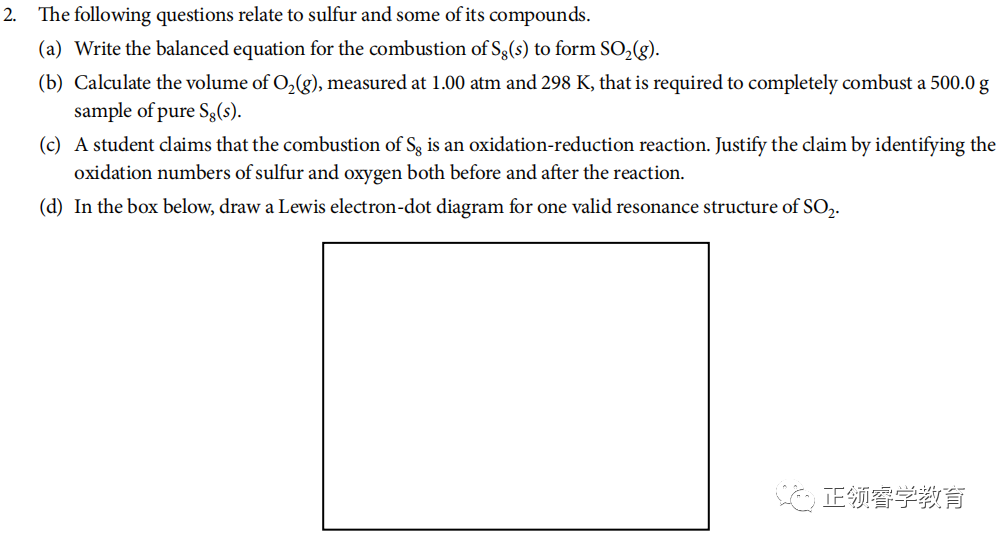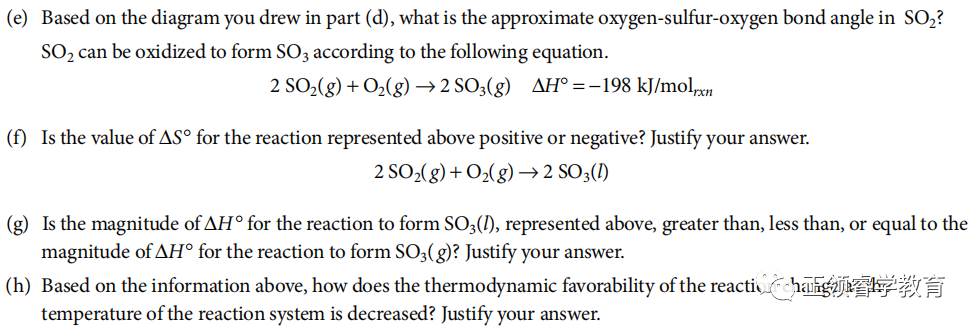(a)  S8(g) + 8 O2(s) → 8 SO2(g)

(b) Use chemical equation to carry out calculations: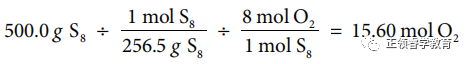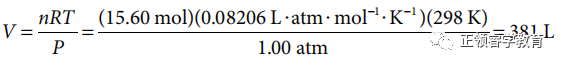(c)  Oxidation numbers before the reaction: S = 0, O = 0

Oxidation numbers after the reaction: S = +4, O = −2

(d)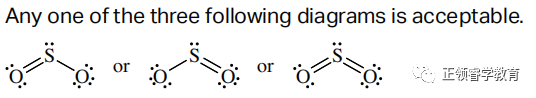(e)  120o.

(f)  Negative, because the reactants are three moles of gas, but the products are only two moles of gas.

(g)  Greater, because the enthalpy of SO3 (l) is lower than the enthalpy of SO3 (g) (by an amount equal to the enthalpy of vaporization of SO3 (l)), which makes the enthalpy change of the reaction greater.

(h) ΔG= ΔH− TΔS. Assuming that both ΔH and ΔS remain constant, as the value of T decreases, the smaller in value the term TΔS becomes, making the term ΔH−TΔS more negative. Thus ΔG becomes more negative, which increases the thermodynamic favorability of the reaction.AMC8辅导、欧几里得竞赛辅导、Physics Bowl物理碗辅导、USABO辅导、BBO辅导、CCC计算机辅导、USACO辅导、UKCHO辅导热门上半年热门赛事
AMC8辅导、欧几里得竞赛辅导、Physics Bowl物理碗辅导、USABO辅导、BBO辅导、CCC计算机辅导、USACO辅导、UKCHO辅导热门上半年热门赛事### 留学生美国看病常用英文有哪些？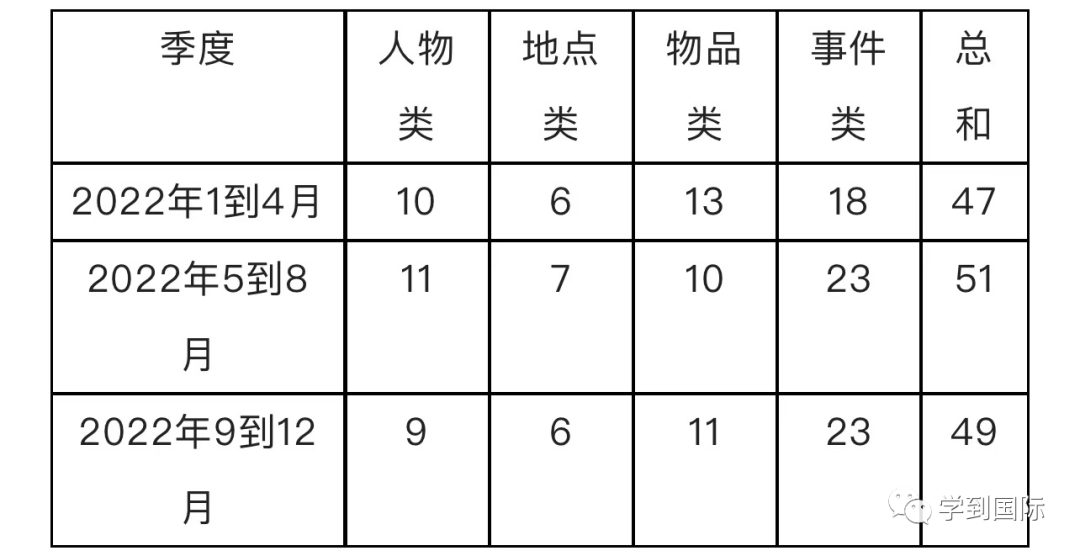5. ### 是否可以使用智能AI写IB论文？可能性后果是什么？老师微信